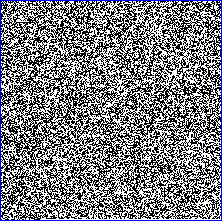# Cryptographic Pseudorandom Number Generator

This PseudoRandom Number Generator (PRNG) allows you to generate small (minimum 1 byte) to large (maximum 16384 bytes) pseudo-random numbers for cryptographic purposes.

It is called pseudorandom because the generated numbers are not true random numbers but are generated using a mathematical formula. This PRNG produces a sequence of bits that "appears" to be random, i.e., the output is statistically indistinguishable from random values.

This tool uses two methods to generate cryptographic pseudorandom numbers depending if your browser supports it.
Each randomly generated byte can be separated by a space, dash or colon and can be encoded in the following formats:
• Hexadecimal (Value always formatted with 2 digits, e.g.: 0d)
• Decimal (Value has 1 to 3 digits, e.g.: 29)
• Octal (Value always formatted with 3 digits, e.g.: 057)
• Binary (Value always formatted with 8 digits, e.g.: 00101101)
For each generated pseudorandom number a bitmap is created to visualize its randomness, for example:## Cryptographic Pseudorandom Number Generator input:

 Number of bytes *:Max 16384 bytes. Encoding *:Hexadecimal Decimal Octal Binary    Uppercase Separator *:none space " " dash "-" colon ":" * = required